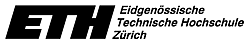# 263-5001-00L Introduction to finite elements and sparse linear system solving Fall semester 2017

Friday 8-11, CAB G56
4 ECTS credits

The finite element(FE) method is the method of choice for (approximately) solving partial differential equations on complicated domains. In the first third of the lecture, we give an introduction to the method. The rest of the lecture will be devoted to methods for solving the large sparse linear systems of equations that are typical for the FE method. We will consider both direct and iterative methods with emphasis on the latter.

## News

• First lecture is on September 22.

## Content

• I. THE FINITE ELEMENT METHOD
• (1) Introduction, model problems.
• (2) 1D problems. Piecewise polynomials in 1D.
• (3) 2D problems. Triangulations. Piecewise polynomials in 2D.
• (4) Variational formulations. Galerkin finite element method. Implementation aspects.
• (5) Theory of errors/error estimation. Adaptive mesh refinement.
• (6) Some problems beyond the Poisson equation.
• II. DIRECT SOLVERS FOR SPARSE LINEAR SYSTEMS
• (7) LU and Cholesky decompositions.
• (8) Sparse matrices. Storage schemes.
• (9) Fill-reducing orderings.
• III. ITERATIVE SOLVERS FOR SPARSE LINEAR SYSTEMS
• (10) Stationary iterative methods, preconditioning.
• (11) Preconditioned conjugate gradient algorithm (PCG).
• (12) Krylov space methods for nonsymmetric systems:
Generalized Minimal RESidual (GMRES) algorithm.
• (13) Incomplete factorization preconditioning.
• (14) Multigrid preconditioning.
• (15) Indefinite problems (SYMMLQ, MINRES).
• (16) Nonsymmetric Lanczos iteration based methods: Bi-CG, QMR, CGS, BiCGstab.

## Literature

1. M. G. Larson, F. Bengzon: The Finite Element Method: Theory, Implementation, and Applications. Springer, Heidelberg, 2013.
2. H. Elman, D. Sylvester, A. Wathen: Finite elements and fast iterative solvers (2nd ed.). Oxford University Press,  Oxford,  2014.
Chapter 1 of 1st edition.
3. Y. Saad:Iterative methods for sparse linear systems (2nd ed.). SIAM, Philadelphia, 2003.
4. T. Davis: Direct Methods for Sparse Linear Systems. SIAM,  Philadelphia, 2006.
5. T. Davis, S. Rajamanickam, W. Sid-Lakhdar: A survey of direct methods for sparse linear systems. Acta Numerica, 25, pp. 383-566 (2016).
6. V. Dolean, P. Jolivet, F. Nataf: An Introduction to Domain Decomposition Methods: Algorithms, Theory, and Parallel Implementation. SIAM,  Philadelphia,  2015.
7. H.R. Schwarz: Die Methode der finiten Elemente (3rd ed.). Teubner,  Stuttgart,  1991.